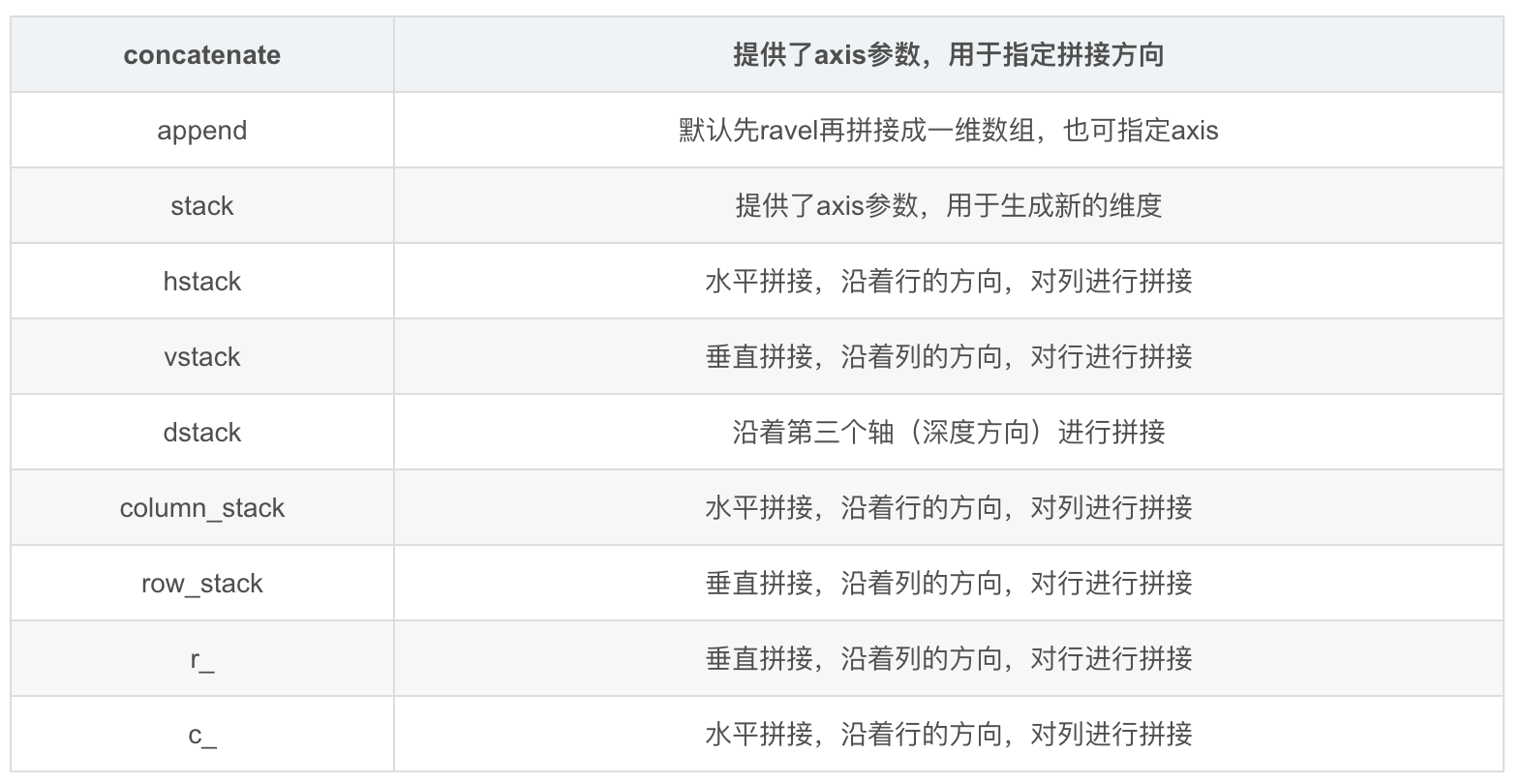### 数据类型转换

#### (1) DataFrame转换为array

• 使用DataFrame中的values方法
• 使用Numpy中的array方法

### 数据合并、拼接

#### （1） DataFrame的合并

• pandas的merge函数

`df = pd.merge(df1, df2, how='left', on='id')`

`how='left'` 表示以df1为基准数据，`on='id'`表示合并时的索引ID

• pandas的concat函数

`df = pd.concat(df1,df2, axis )`, axis=1表示列拼接，axis=0表示行拼接

#### (2) Array的合并• concatenate

• stack

`np.stack(())`合并后的数据是多个数组，纬度增加。参数axis默认值为0.

• hstack：水平合并, 数组纬度没变
• vstack：垂直合并，数组纬度没变